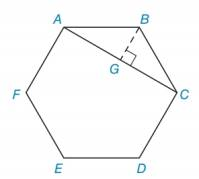Chapter 7.3, Problem 30EElementary Geometry For College St...

7th Edition
Alexander + 2 others
ISBN: 9781337614085

Solutions

Chapter
SectionElementary Geometry For College St...

7th Edition
Alexander + 2 others
ISBN: 9781337614085
Textbook Problem

Given regular hexagon ABCDEF with each side of length 6, find the length of diagonal A C ¯ . (Hint: With G on A C ¯ , draw B G ¯   ⊥   A C ¯ .)To determine

To find:

The length of the diagonal AC¯.

Explanation

Approach:

The measure of interior angle of regular polygon of n sides is given by (n2)180n

Calculation:

Consider the given figure.

Interior angles of regular hexagon =(62)1806=120

Therefore, ABC=120

Draw a perpendicular BG¯ from B to AC¯ at G.

BG¯ will bisect and AC¯andABC

<

Still sussing out bartleby?

Check out a sample textbook solution.

See a sample solution

The Solution to Your Study Problems

Bartleby provides explanations to thousands of textbook problems written by our experts, many with advanced degrees!

Get Started

Find more solutions based on key concepts